# Objectives Solving Linear Equations Solving Quadratic Equations Other

• Slides: 37Objectives ► Solving Linear Equations ► Solving Quadratic Equations ► Other Types of Equations 1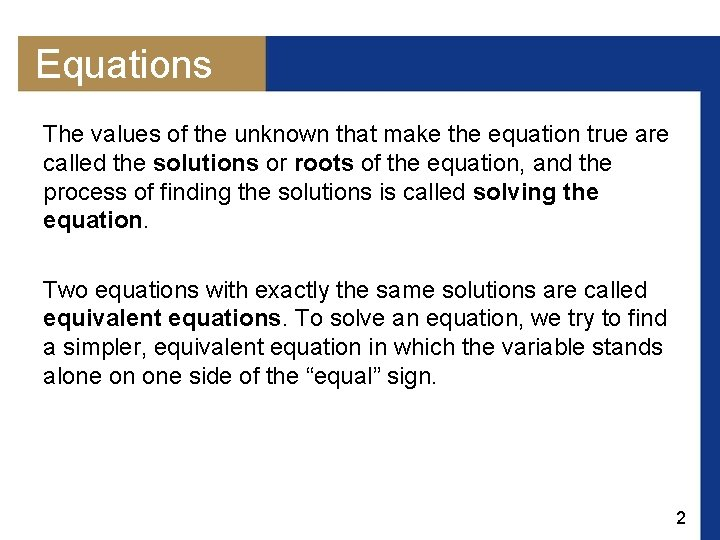Equations The values of the unknown that make the equation true are called the solutions or roots of the equation, and the process of finding the solutions is called solving the equation. Two equations with exactly the same solutions are called equivalent equations. To solve an equation, we try to find a simpler, equivalent equation in which the variable stands alone on one side of the “equal” sign. 2Equations 3Solving Linear Equations the difference between linear and nonlinear equations. 4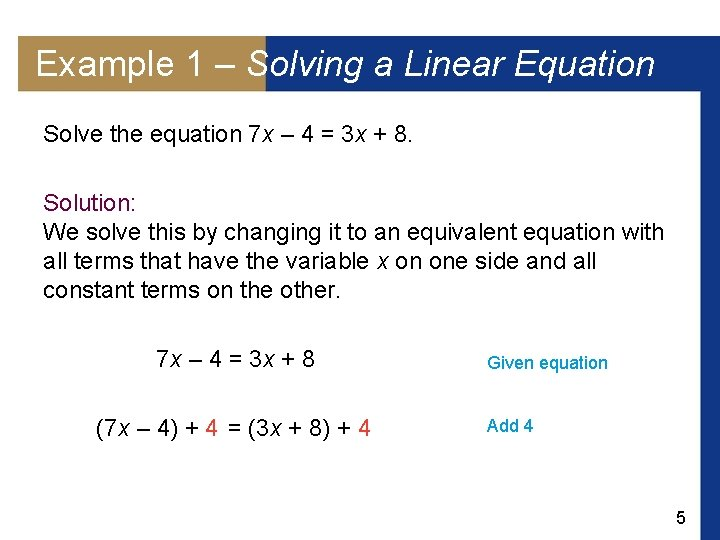Example 1 – Solving a Linear Equation Solve the equation 7 x – 4 = 3 x + 8. Solution: We solve this by changing it to an equivalent equation with all terms that have the variable x on one side and all constant terms on the other. 7 x – 4 = 3 x + 8 (7 x – 4) + 4 = (3 x + 8) + 4 Given equation Add 4 5Example 1 – Solution 7 x = 3 x + 12 7 x – 3 x = (3 x + 12) – 3 x 4 x = 12 cont’d Simplify Subtract 3 x Simplify Multiply by x=3 Simplify 6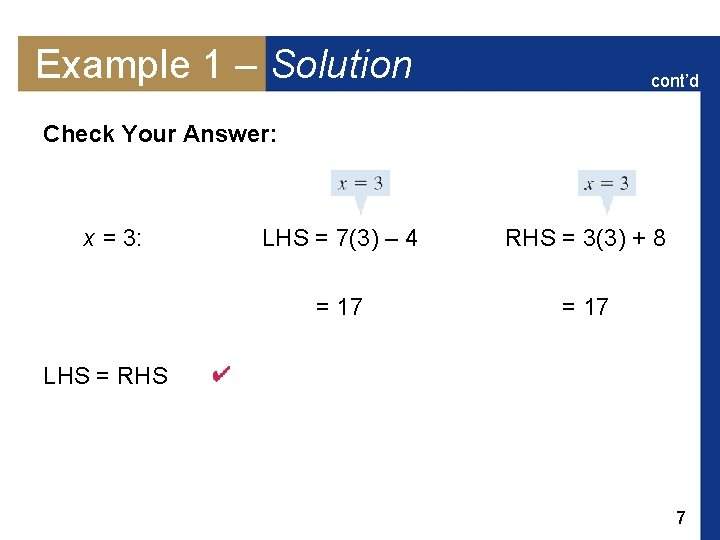Example 1 – Solution cont’d Check Your Answer: x = 3: LHS = 7(3) – 4 RHS = 3(3) + 8 = 17 LHS = RHS 7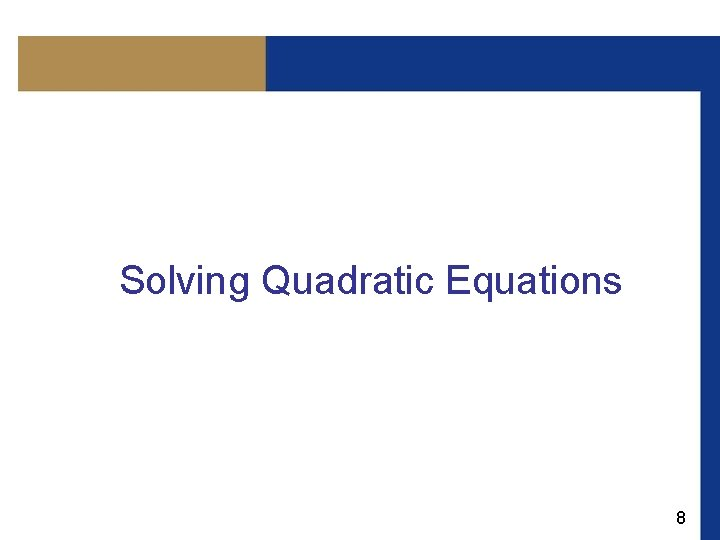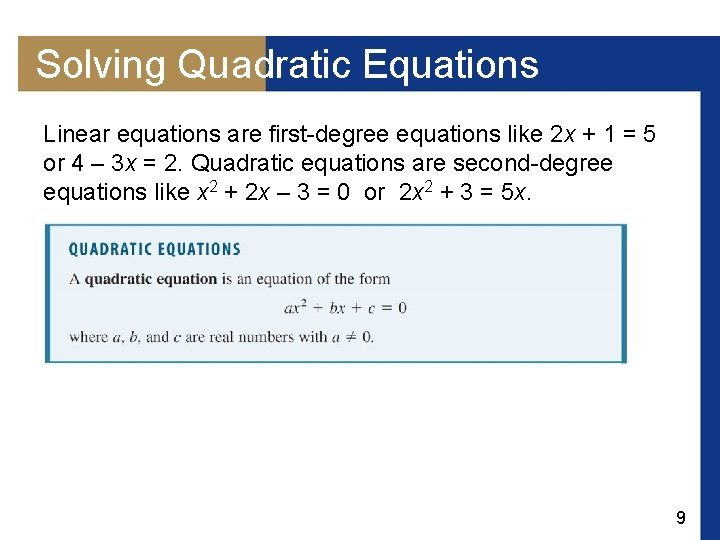Solving Quadratic Equations Linear equations are first-degree equations like 2 x + 1 = 5 or 4 – 3 x = 2. Quadratic equations are second-degree equations like x 2 + 2 x – 3 = 0 or 2 x 2 + 3 = 5 x. 9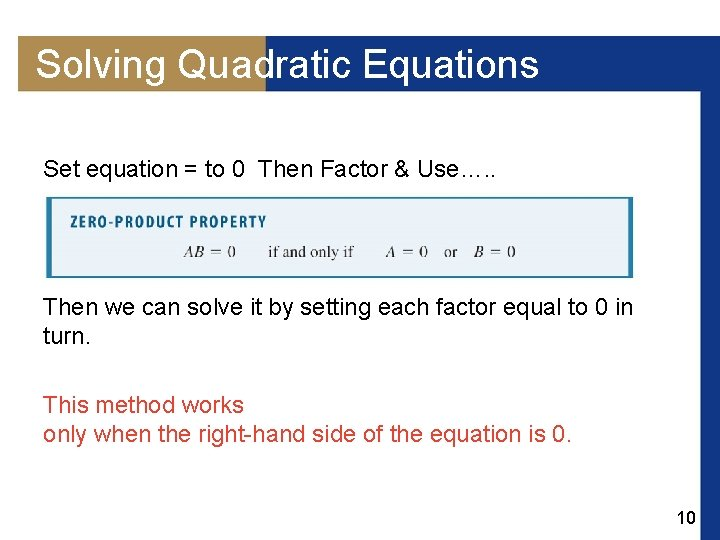Solving Quadratic Equations Set equation = to 0 Then Factor & Use…. . This means that if we can factor the left-hand side of a, Then we can solve it by setting each factor equal to 0 in turn. This method works only when the right-hand side of the equation is 0. 10Example 4 – Solving a Quadratic Equation by Factoring Solve the equation x 2 + 5 x = 24. Subtract 24 Solution: rewrite the equation so the right-hand side is 0. x 2 + 5 x – 24 = 0 (x – 3)(x + 8) = 0 x– 3=0 x=3 or x+8=0 x = – 8 And Check your answers!!! Factor Zero-Product Property Solve 11Example 5 – Solving Simple Quadratics (a) x 2 = 5 Solution: (a) (b) (x – 4)2 = 5 (b)Take the square root of each side of the equation. (x – 4)2 = 5 Take the square root 12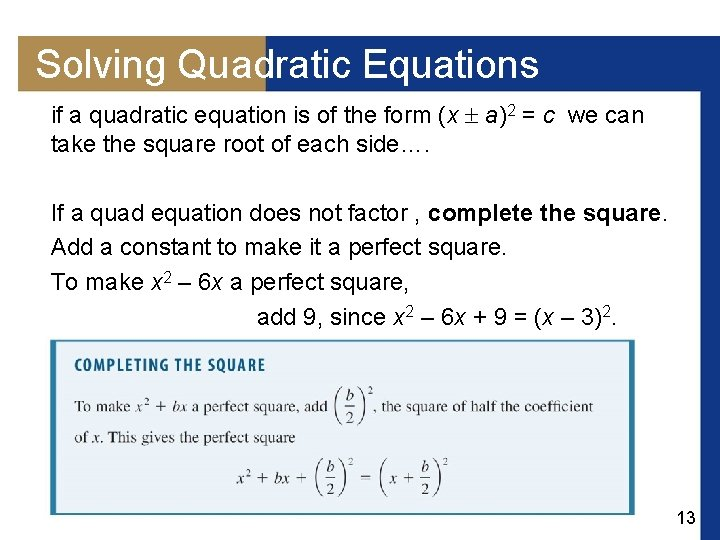Solving Quadratic Equations if a quadratic equation is of the form (x a)2 = c we can take the square root of each side…. If a quad equation does not factor , complete the square. Add a constant to make it a perfect square. To make x 2 – 6 x a perfect square, add 9, since x 2 – 6 x + 9 = (x – 3)2. 13Example 6 – Solving Quadratic Equations by Completing the Square Solve each equation. (a) x 2 – 8 x + 13 = 0 (b) 3 x 2 – 12 x + 6 = 0 Solution: (a) x 2 – 8 x + 13 = 0 x 2 – 8 x = – 13 x 2 – 8 x + 16 = – 13 + 16 Given equation Subtract 13 Complete the square: add = 16 Perfect square Take square root Add 4 14Example 6 – Solution 3 x 2 – 12 x + 6 = 0 3 x 2 – 12 x = – 6 cont’d Given equation Subtract 6 Factor 3 from LHS 3(x 2 – 4 x) = – 6 we complete the square by adding (– 2)2 = 4 inside the parentheses. Since everything inside the parentheses is multiplied by 3, this means that we are actually adding 3 4 = 12 to the left side of the equation. 15Example 6 – Solution cont’d Thus, we must add 12 to the right side as well. 3(x 2 – 4 x + 4) = – 6 + 3 4 3(x – 2)2 = 6 (x – 2)2 = 2 Complete the square: add 4 Perfect square Divide by 3 Take square root Add 2 16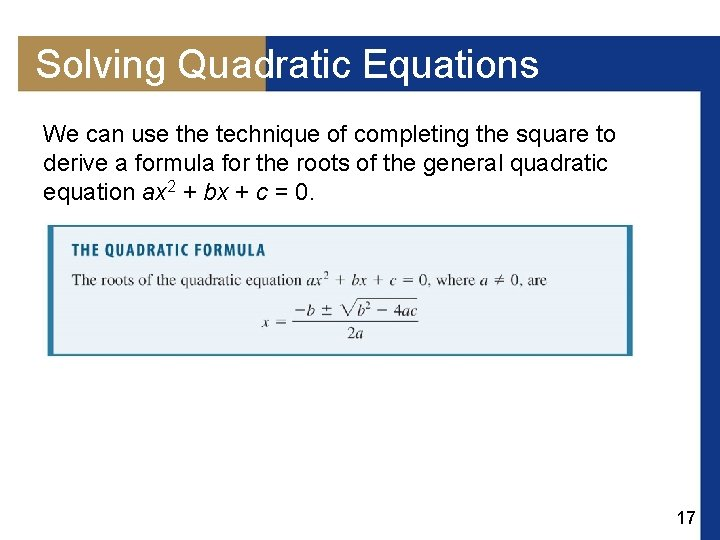Solving Quadratic Equations We can use the technique of completing the square to derive a formula for the roots of the general quadratic equation ax 2 + bx + c = 0. 17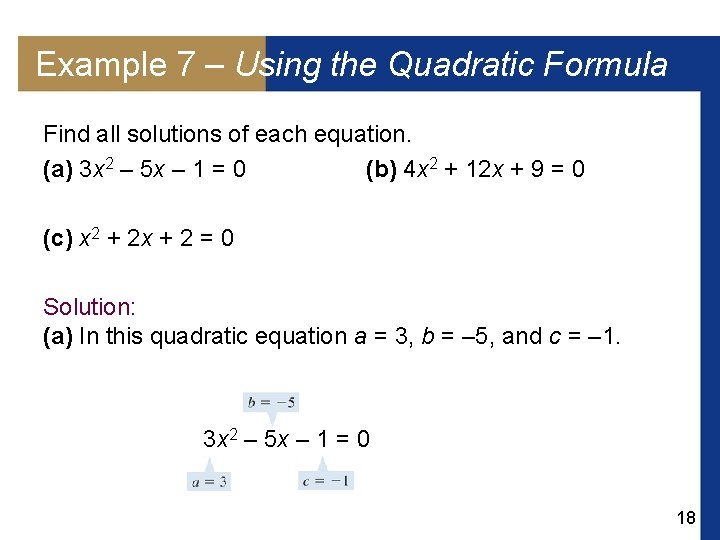Example 7 – Using the Quadratic Formula Find all solutions of each equation. (a) 3 x 2 – 5 x – 1 = 0 (b) 4 x 2 + 12 x + 9 = 0 (c) x 2 + 2 x + 2 = 0 Solution: (a) In this quadratic equation a = 3, b = – 5, and c = – 1. 3 x 2 – 5 x – 1 = 0 18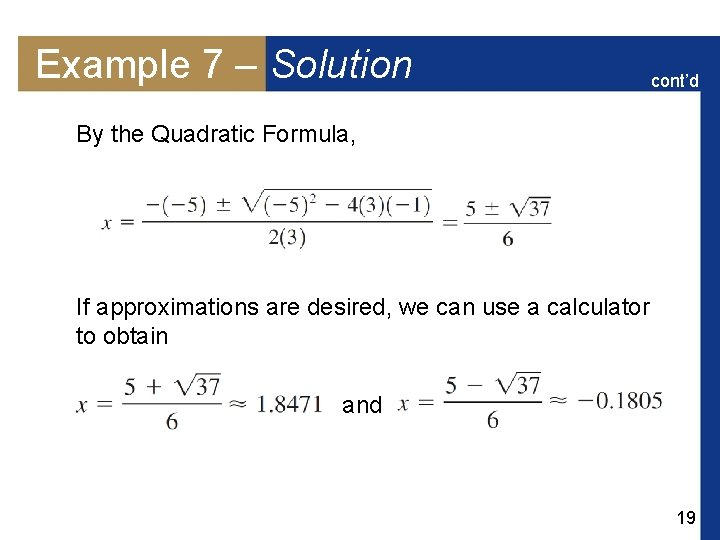Example 7 – Solution cont’d By the Quadratic Formula, If approximations are desired, we can use a calculator to obtain and 19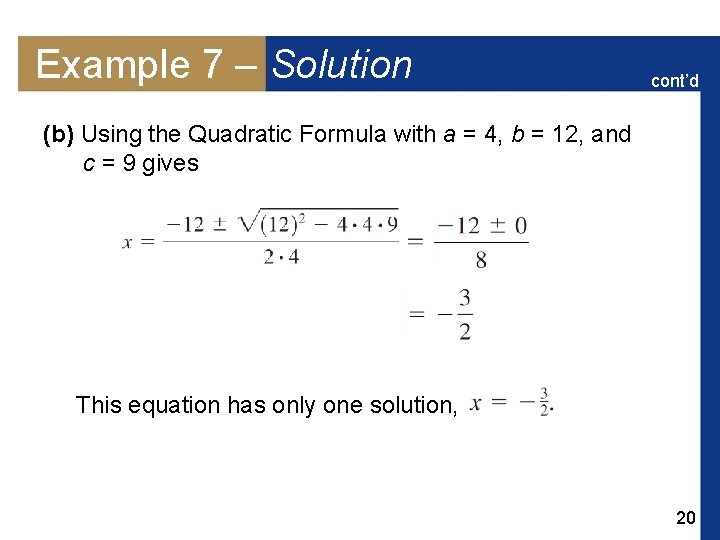Example 7 – Solution cont’d (b) Using the Quadratic Formula with a = 4, b = 12, and c = 9 gives This equation has only one solution, 20Example 7 – Solution cont’d (c) Using the Quadratic Formula with a = 1, b = 2, and c = 2 gives 21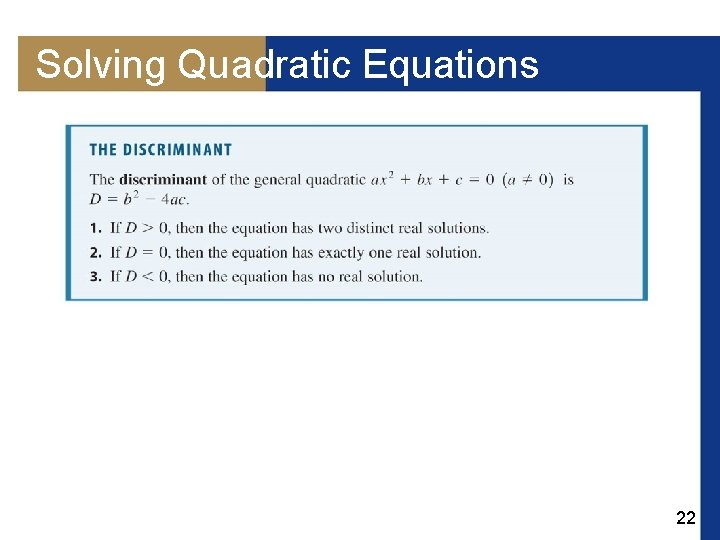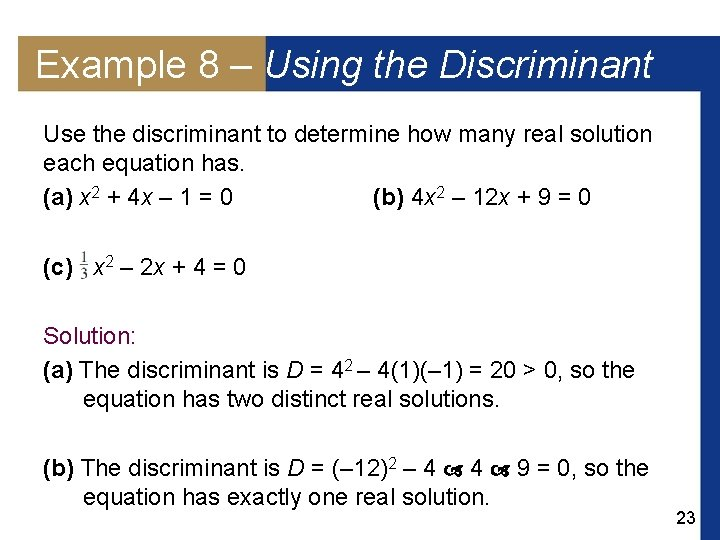Example 8 – Using the Discriminant Use the discriminant to determine how many real solution each equation has. (a) x 2 + 4 x – 1 = 0 (b) 4 x 2 – 12 x + 9 = 0 (c) x 2 – 2 x + 4 = 0 Solution: (a) The discriminant is D = 42 – 4(1)(– 1) = 20 > 0, so the equation has two distinct real solutions. (b) The discriminant is D = (– 12)2 – 4 4 9 = 0, so the equation has exactly one real solution. 23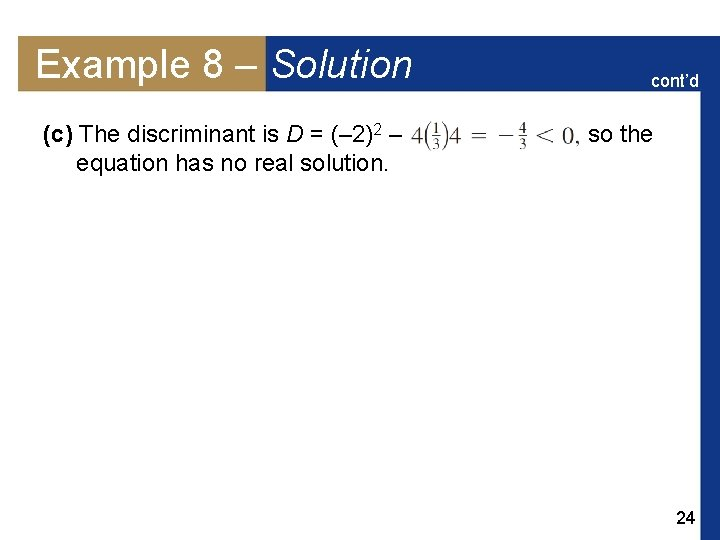Example 8 – Solution (c) The discriminant is D = (– 2)2 – equation has no real solution. cont’d so the 24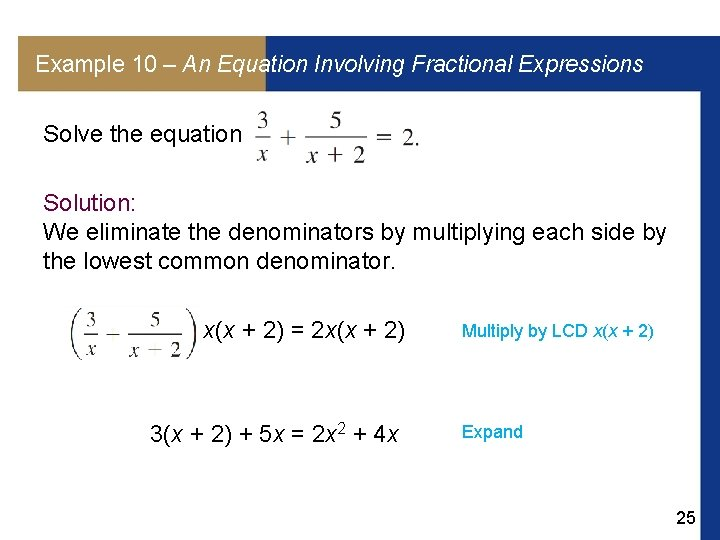Example 10 – An Equation Involving Fractional Expressions Solve the equation Solution: We eliminate the denominators by multiplying each side by the lowest common denominator. x(x + 2) = 2 x(x + 2) 3(x + 2) + 5 x = 2 x 2 + 4 x Multiply by LCD x(x + 2) Expand 25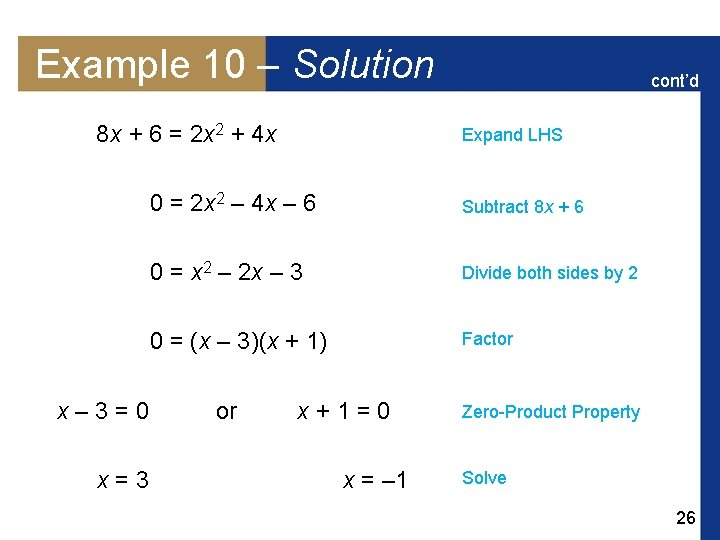Example 10 – Solution 8 x + 6 = 2 x 2 + 4 x x– 3=0 x=3 cont’d Expand LHS 0 = 2 x 2 – 4 x – 6 Subtract 8 x + 6 0 = x 2 – 2 x – 3 Divide both sides by 2 0 = (x – 3)(x + 1) Factor or x+1=0 x = – 1 Zero-Product Property Solve 26Example 10 – Solution cont’d We must check our answers because multiplying by an expression that contains the variable can introduce extraneous solutions. From Check Your Answers we see that the solutions are x = 3 and – 1. Check Your Answers: x = 3: LHS = =1+1=2 RHS = 2 LHS = RHS 27Example 10 – Solution cont’d x = – 1: LHS = = – 3 + 5 = 2 RHS = 2 LHS = RHS 28Example 11 – An Equation Involving a Radical Solve the equation Solution: To eliminate the square root, we first isolate it on one side of the equal sign, then square: Subtract 1 (2 x – 1)2 = 2 – x 4 x 2 – 4 x + 1 = 2 – x Square each side Expand LHS 29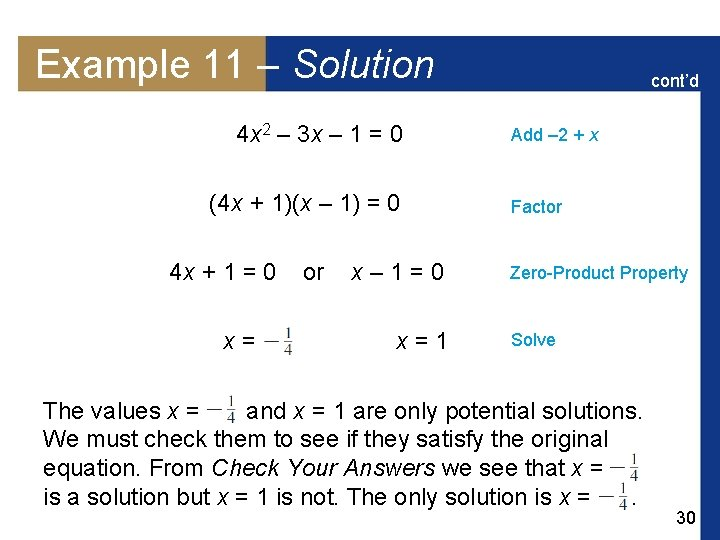Example 11 – Solution 4 x 2 – 3 x – 1 = 0 (4 x + 1)(x – 1) = 0 4 x + 1 = 0 x= or x– 1=0 x=1 cont’d Add – 2 + x Factor Zero-Product Property Solve The values x = and x = 1 are only potential solutions. We must check them to see if they satisfy the original equation. From Check Your Answers we see that x = is a solution but x = 1 is not. The only solution is x =. 30Example 11 – Solution cont’d Check Your Answers: LHS = RHS = LHS = RHS 31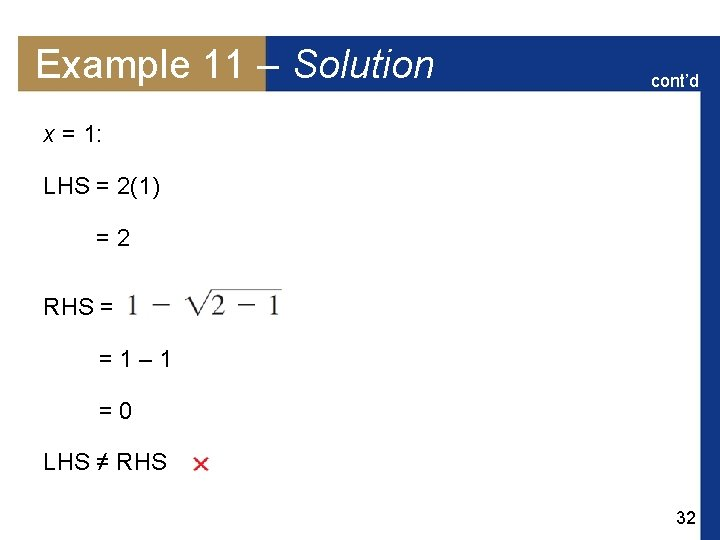Example 11 – Solution cont’d x = 1: LHS = 2(1) =2 RHS = =1– 1 =0 LHS ≠ RHS 32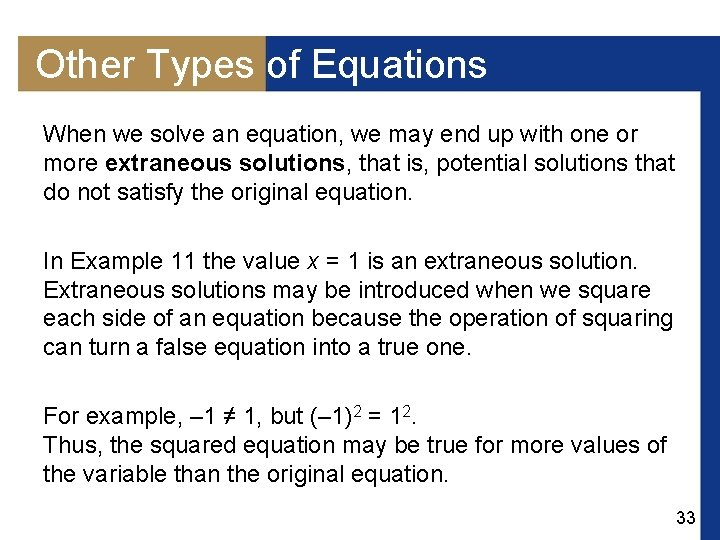Other Types of Equations When we solve an equation, we may end up with one or more extraneous solutions, that is, potential solutions that do not satisfy the original equation. In Example 11 the value x = 1 is an extraneous solution. Extraneous solutions may be introduced when we square each side of an equation because the operation of squaring can turn a false equation into a true one. For example, – 1 ≠ 1, but (– 1)2 = 12. Thus, the squared equation may be true for more values of the variable than the original equation. 33Other Types of Equations That is why you must always check your answers to make sure that each satisfies the original equation. An equation of the form a. W 2 + b. W + c = 0, where W is an algebraic expression, is an equation of quadratic type. We solve equations of quadratic type by substituting for the algebraic expression, as we see in the next example. 34Example 12 – A Fourth-Degree Equation of Quadratic Type Find all solutions of the equation x 4 – 8 x 2 + 8 = 0. Solution: If we set W = x 2, then we get a quadratic equation in the new variable W: (x 2)2 – 8 x 2 + 8 = 0 W 2 – 8 W + 8 = 0 Write x 4 as (x 2)2 Let W = x 2 35Example 12 – Solution cont’d Quadratic Formula W = x 2 Take square roots 36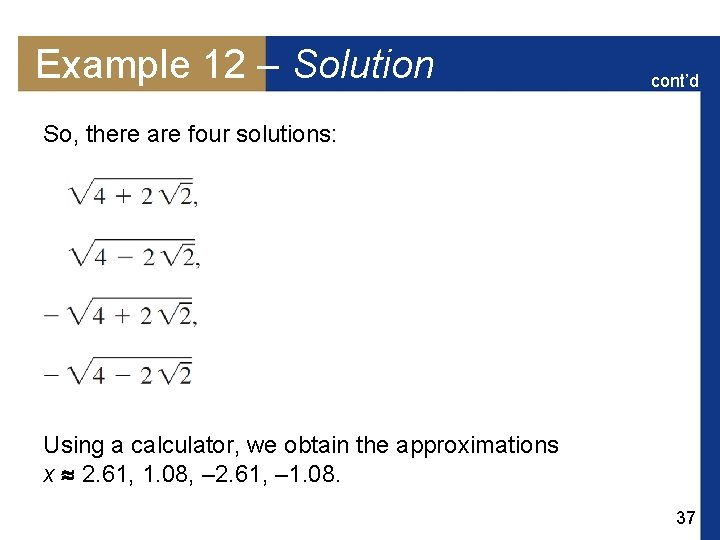Example 12 – Solution cont’d So, there are four solutions: Using a calculator, we obtain the approximations x 2. 61, 1. 08, – 2. 61, – 1. 08. 37